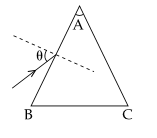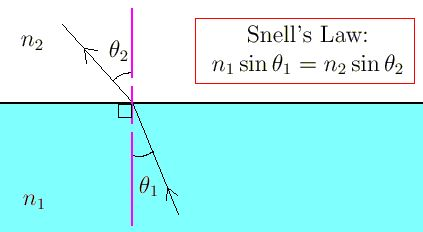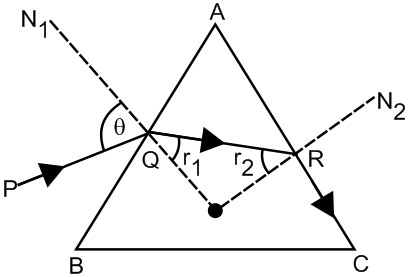#### Monochromatic light is incident on a glass prism of angle A. If the refractive index of the material of the prism is, a ray, incident at an angle, on the face AB would get transmitted through the face AC of the prism provided :Option 1)Option 2)Option 3)Option 4)As we learnt in

Relation between angle of incidence and angle of refaction -- whereinrefractive index of medium of incidence.refractive index of medium where rays is refracted.angle of incidence.angle of refraction.Where r2 = Cwhere c = critical angleApplying Snell's law at R(i)

Snell's law at Q(ii)(iv)                    (iii)From equation (iii) and (iv)Correct option is 1.

Option 1)This is the correct option.

Option 2)This is an incorrect option.

Option 3)This is an incorrect option.

Option 4)This is an incorrect option.

##### Posted by